## Wednesday, September 16, 2015

### Stack Machine generated Textures

This is something I made Just a few weeks ago. You feed it Strings and it gives you Textures, It's not an easy thing to use but I find it quite fun.  The goal was to provide the ability to include small textures inside code.

The images are generated in a reverse polish notation stack expression.  The expression is calculated for every pixel in the image, with x and y indicating the current pixel location within the image.   The codes that stackie understands are:

x : push x (x is in range 0...1)
y : push y (y is in range 0...1)
0...9 : push constant 0...9
P : push PI

d : duplicate top item on the stack
: : swap top two items on the stack
; : swap top and third items on the stack

s : sin
c : cos
q : sqrt
~ : abs
! : flip        1-a                  implemented as  push(1-pop())
# : round to nearest integer
? : threshold  ( value<=0 becomes 0, value>0 becomes 1 );

p : perlin noise (using top two stack values)
w : wraparound perlin noise using three stack valuesx,y,size
W : wraparound perlin noise using 4 stack values x,y,x_size, y_size

a : atan2
- : subtract
* : multiply
/ : divide
^ : pow
> : max
< : min

While the final strings can look incomprehensible, writing a texture code is easier than it seems.  You can build it up by constructing simple pieces and mixing them together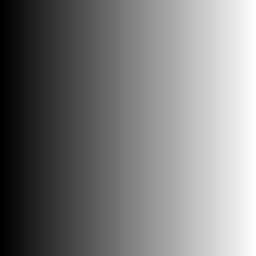x y
x and y make simple vertical and horizontal gradients.x! y!
! inverts the value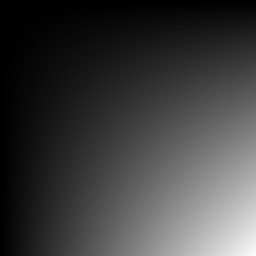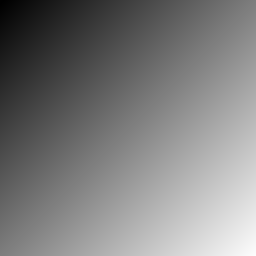xy* xy+2/
Multiply two gradients together, or add them together and divide by 2 for a simple average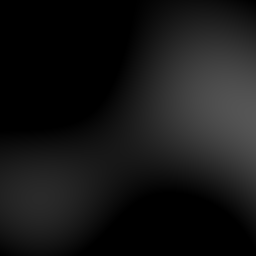xyp x8*y8*p1+2/
p generates perlin noise. For higher frequency noise multiply the x and y values by a scale factorx9^ y9^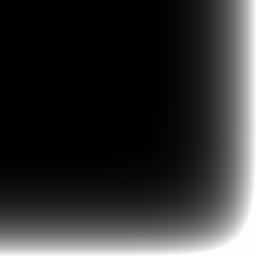x9^ y9^ + x9^ y9^ + x!9^ y!9^ + +

By combining simple sequences together you can construct  more complex images.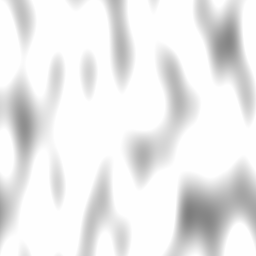x8* y3* p 1+ x9^ x!9^ + y! + +x8* y3* p 1+ x9^ x!9^ + y! + - Palette xy!1+*
Have a play around with it in the iframe

Stackie is on github